# Previous Years GATE Questions on Joining Process 2005-2011

## Solved GATE Questions on Joining Process

(A) 1480 A

(B) 3300 A

(C) 4060 A

(D) 9400 A

GATE-ME-2005

Hint 1. (Ans C)

### Question 2. The strength of a brazed joint

(A) Decrease with increase in gap between the two joining surfaces

(B) Increase with increase in gap between the two joining surfaces

(C) Decrease up to certain gap between the two joining surfaces beyond which it increases

(D) Increases up to certain gap between the two joining surfaces beyond which it decreases

GATE-ME-2005

Hint 2. (Ans D)

Strength increases upto some certain gap and beyond this strength decreases.

### Question 3. In an arc welding process, the voltage and current are 25 V and 300 A respectively. The arc heat transfer efficiency is 0.85 and welding speed is 8 mm/sec. The net heat input (in J/mm) is :

(A) 64

(B) 797

(C) 1103

(D) 79700

GATE-ME-2006

Hint 3. (Ans B)

Power generated=VIHeat input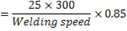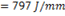### Question 4. Two metallic sheets, each of 2.0 mm thickness, are welded in a lap joint configuration by resistance spot welding at a welding current of 10 kA and welding time of 10 milli second. A spherical fusion zone extending up to the full thickness of each sheet is formed. The properties of the metallic sheets are given as:

Ambient temperature = 293 K

Melting temperature = 1793 K

Density =7000 kg/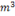Latent heat of fusion=300 kJ/kg

Specific heat =800 J/kg K

Assume : (i) contact resistance along sheet—sheet interface is 500 micro-ohm and along electrode—sheet interface is zero; (ii) no conductive heat loss through the bulk sheet materials; and (iii) the complete weld fusion zone is at the melting temperature.

(A) 50.37

(B) 60.37

(C) 70.37

(D) 80.37

GATE-ME-2007

Hint 4. (Ans C)

Heat generated due to current,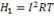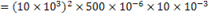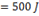Heat required for melting,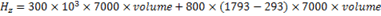Since fusion zone is spherical in shape, therefore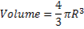Where r=t=thickness of sheet=2mm

Melting efficiency=70.35 %

### Question 5. A direct current welding machine with a linear power source characteristic provides open circuit voltage of 80 V and short circuit current of 800 A. During welding the machine, the measured arc current is 500 A corresponding to an arc length of 5.0 mm and the measured arc current is 460 A corresponding to an arc length of 7.0 mm. the linear voltage (E)-arc length (L) characteristic of the welding arc can be given as (where E is in volt and L is in mm)

(A) E=20+2L

(B) E=20+8L

(C) E=80+2L

(D) E=80+8L

GATE-ME-2007

Hint 5. (Ans A)

From power–source characteristics,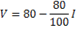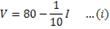Arc voltage characteristics,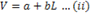Equating (i) and (ii), we get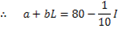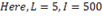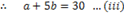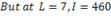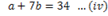From equation (iii) and (iv), we get b=2 a=20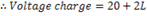### Question 6. Which one of the following is a solid state joining process?

(A) Gas tungsten arc welding

(B) Resistance spot welding

(C) Friction welding

(D) Submerged arc welding

GATE-ME-2007

Hint 6. (Ans C)

Friction welding is clearly solid state welding.

### Question 7. In arc welding of a butt joint, the welding speed is to be selected such that highest cooling rate is achieved. Melting efficiency and heat transfer efficiency are 0.5 and 0.7 respectively.the artea of the weld cross-section is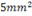and the unit energy required to melt the metal is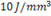. If the welding power is 2 kW, the welding speed in mm/s is closest to

(A) 4

(B) 14

(C) 24

(D) 34

GATE-ME-2008

Hint 7. (Ans B)

Welding power applied=Heating power needed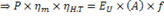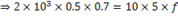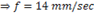### Question 8. Two pipes of inner diameter 100 mm and outer diameter 110 mm each are joined by flash welding using 30 V power supply. At the interface, 1 mm of material melts from each pipe which has a resistance of 42.4 Ω. If the unit melt energy is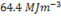, then time required for welding (in s) is

(A) 1

(B) 5

(C) 10

(D) 20

GATE-ME-2010

Hint 8. (Ans C)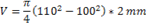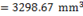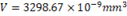Heat energy required for welding.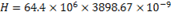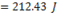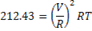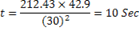### Question 9. Which one of the following welding processes used non–consumable electrode?

(A) Gas metal arc welding

(B) Submerged arc welding

(C) Gas tungsten arc welding

(D) Flux coated arc welding

GATE-ME-2011

Hint 9. (Ans C)

Gas tungsten arc welding uses a non—consumable electrode made of tungsten.

### Question 10. Match the correct pairs.

 Processes Characteristics/Applications P. Friction welding 1. non—consumable electrode Q. Gas metal arc welding 2. joining of thick plates R. Tungsten inert gas welding 3. consumable electrode wire S. electroslag welding 4. joining of cylindrical dissimilar materials

(A) P—4,  Q—3,   R—1,   S—2

(B) P—4,  Q—2,   R—3,   S—1

(C) P—2,  Q—3,   R—4,   S—1

(D) P—2,  Q—4,   R—1,   S—3

GATE-ME-2011

Hint 10. (Ans A)

1. (C)   2. (D)   3. (B)   4. (C)   5. (A)   6. (C)   7. (B)   8. (C)   9. (C)   10. (A)

### 6 Responses to “Previous Years GATE Questions on Joining Process 2005-2011”

1.#### Vinay mani tripathi

Well Colletion .and study material

2.Thanks for the appreciation

3.#### M G R

Really good collection and great hard Work. I have request if you can upload the later question paper for all subjects and topics it would be of great help.

4.#### amol

Thanks, great work

5.6.